Plus Blog

April 1, 2015You do modular arithmetic several times every day when you are thinking about time. Imagine, for example, you're going on a train trip at 11pm that lasts three hours. What time will you arrive? Not at 11+3 = 14 o'clock, but at 2 o'clock in the morning. That's because, on a 12-hour clock, you start counting from the beginning again after you get to 12. (On a 24-hour clock, you start again after you get to 24.) So, on a 12-hour clock you have:

4 + 9 = 1,
7 + 7 = 2,
5 + 12 = 5,

and so on. When you are subtracting hours, you do the same but backwards:

4 - 7 = 9
1 - 11 = 2
6 - 12 = 6.

You could play the same game using other numbers, apart from 12 and 24, to define your cycle. For example, in modular arithmetic modulo 5 you have

4 + 2 = 1
3 + 4 = 2
1 - 4 = 2
3 - 5 = 3

These sums can be a little tedious to work out if you're counting on your fingers, but luckily there is a general method. Let's say you're doing arithmetic modulo some natural number p and you're looking at some other natural number x. To find the value of x modulo p (the value of x on a clock with p hours), compute the remainder when dividing x by p: that's your result.

This also works when x is negative (noting that the remainder is defined to be always positive). For example, for p = 12 and x = -3 we have

-3 = (-1) x 12 + 9,

so the remainder is 9. Therefore -3 modulo 12 is equal to 9. (If you use the modulus function in some computer languages you have to be a little careful though, as some return a different value for negative numbers.)

If you want to add or subtract two numbers module some natural number p, you simply work out the result, call it x, in ordinary arithmetic and then find the value of x modulo p.

There is clearly something very cyclical about modular arithmetic. Whatever number p defines your arithmetic, you can think of it as counting forward or backward in clock with p hours. To put this in technical maths language, modular arithmetic modulo p gives you a cyclic group of order p. You can find out more about group theory in this article and about modular arithmetic on our sister site NRICH.

March 27, 2015One way to start getting your head around groups, those slightly scary abstract structures that belong to the field of algebra, is to think of a 12-hour clock. You can add hours on this clock, for example

2 o'clock + 4 hours = 6 o'clock
8 o'clock + 5 hours = 1 o'clock
3 o'clock + 12 hours = 3 o'clock

and so on. One thing to notice here is that however many hours you add, the answer is always going to be a number between 1 and 12. In this sense, addition on a 12-hour clock is closed: it never gets you out of the 1 to 12 land.

Another thing to notice is that adding 12 hours gets you back to where you started (it's the same as doing nothing): for any starting time a, we have

a + 12 = a.

This is why you could equally well write 0 for the number 12 in our clock-world.

A third interesting feature is that when you have added some number a of hours, you can get back to where you started by adding another 12 - a hours. For example, starting at 1 o'clock and adding 7 gives

1 + 7 = 8.

and then adding another 12 - 7 = 5 hours gives

8 + 5 = 1.

So adding a and then 12 - a is the same as doing nothing (or, equivalently, adding 12).

Taking a small step into abstraction, we can describe our 12-hour arithmetic as follows. We have a set S (which consists of the numbers 1 to 12) and a binary operation that combines any two elements of S to give a third (the operation is addition modulo 12 in our example). The set and the operation satisfy the following three rules:

• Whenever you add two elements of the set S the result is also an element of S (addition is closed).
• There is an element of S (in our case the number 12), such that when you add that element to any other element a of S, the result is a. This element is called the identity of S.
• For every element a of S there is another element b of S, so that a + b is equal to the identity of S. The element b is called the inverse of a. (In the clock example the inverse of a is 12 - a.)

There is also a fourth rule satisfied by our set and operation:

• For three elements a, b and c of S we have (a + b) + c = a + (b + c). In other words, it doesn't matter whether you add the third number to the sum of the first two, or whether add the sum of the last two numbers to the first number.

Move the slider to rotate the 12-gon.

There are many other structures that also satisfy these rules. As an example, think of a regular 12-gon, as the one pictured on the left. You can rotate this shape clockwise around its centre by 30 degrees and what you end up with is the same shape as the one you started with. The rotation is a symmetry of the 12-gon. Now let our set S consist of all the clockwise rotations through multiples of 30 degrees. So that's 30 degrees, 60 degrees, 90 degrees, etc, all the way up to 330 degrees and, finally, the full turn. (All of these are symmetries of the 12-gon.) Think of adding two rotations as doing one after the other: so the rotation through 60 degrees plus the rotation through 90 degrees equals the rotation through 150 degrees.

This structure forms a group. Addition is closed because when you follow one clockwise rotation through a multiple of 30 degrees by another, the result is also a clockwise rotation through a multiple of 30 degrees. There's also an identity element, namely the rotation through 360 degrees. For every rotation there is an inverse rotation, so that combining the two is the same as doing the identity rotation. For example, the inverse of the rotation through 30 degrees is the rotation through 330 degrees.

In general, a group is a set S of elements together with a binary operation that satisfies the four rules above. Our two examples here are finite groups in that the set S has finitely many elements. But there are also infinite groups. You can try to convince yourself that the set of whole numbers (including the negative ones) with the operation of addition forms an infinite group.

It's interesting to note that two groups can consist of different objects and involve different operations but still have the same structure. By an unbelievably lucky coincidence, the two groups we have seen so far are an example of this. In our clock face example above, adding one hour corresponds to turning the clock hand clockwise through a twelfth of a turn — that's 30 degrees. Adding b hours corresponds to turning the hand through b twelfths of a full turn — that's b x 30 degrees. In fact, the elements of the clock face group (the numbers 1 to 12) behave under their modular addition in exactly the same way as the elements of our 12-gon rotation group do under their own notion of addition. If, in our clock face group, a + b = c, then you know immediately that in the rotation group the rotation through a x 30 degrees followed by the rotation through b x 30 degrees gives the rotation through c x 30 degrees. And vice versa. The two groups are said to be isomorphic. (See here for a technical definition.) Mathematicians often find that a group that arises in one context is isomorphic to a group they know from a very different context.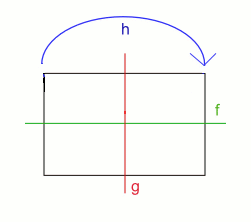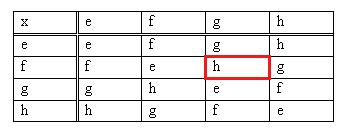This table represents a group called the Klein 4-group.

This is why it can make sense to think of a group, not as made up of rotations or numbers or some other explicit type of objects, but as a collection of abstract objects (we can use letters to denote these objects) that combine in a particular way under the binary operation. You can keep track of how they combine in a table, such as the one on the left. To see the result of the sum f + g, find the cell corresponding to row f and column g. Its entry is h, so f + g = h. The symbol e in this table stands for the group identity.

The group described by this table is known as the Klein 4-group. It's isomorphic to the symmetry group of a rectangle, writing e for the identity symmetry (doing nothing), f for the reflection in the horizontal axis, g for the reflection in the vertical axis and h for the rotation through 180 degrees. Using this abstract representation, you can describe groups that are isomorphic, even if they arise in very different contexts, in one go. That's the power of abstraction!

March 26, 2015

In our Researching the unknown project we explored what physicists at Queen Mary University of London get up to. As part of the project the designer Charles Trevelyan produced these four beautiful posters, which are our images of the week. Feel free to download and print them out, to adorn your classroom, bedroom or wherever you'd like to see them! Simply click on the images to download a pdf.

Click here to see previous images of the week.

March 18, 2015A solar eclipse observed in Australia in 2012. Image: Davidfntau.

On Friday March 20, between around 9:25 GMT and 10:41 GMT, people in the UK will be able to witness a partial eclipse of the Sun! It's the first one since the total eclipse in 1999 and there won't be another one until 2026.

A solar eclipse occurs when the Moon moves in front of the Sun, blotting parts of it out and casting its shadow on the Earth. Some parts on the Earth will see a total eclipse, in which the Moon completely blocks the Sun, giving a great view of its outer atmosphere called the corona.

On Friday only a narrow path a few hundred kilometres wide will see a total eclipse. The only two landmasses in that path are the Faroe Islands and the arctic archipelago of Svalbard. In the UK the Sun will only be partially obscured to varying degrees: Edinburgh will see 93% of the Sun blotted out, Lerwick in the Shetland Isles 97%, and London 85%. In London the eclipse will start at 8:25GMT, peak at 9:31GMT and end at 10:41. Times vary slightly in other parts of the UK, with things happening earlier the further West or South you are.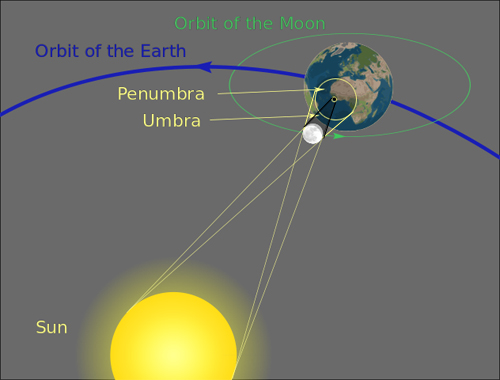The geometry of an eclipse. In the region called the umbra, bounded by the small yellow circle, people see a total eclipse. In the penumbra, bounded by the larger yellow circle, people see a partial eclipse.

But even when the eclipse is only partial, there are still interesting things to look out for. You might see dark spots on the Sun — sunspots — which are caused by the magnetic field of the Sun dimming its light output. You might also be able to observe the outline of the Moon's surface, with its mountains and valleys. If you're somewhere where the sunlight passes through tree foliage, you'll see lots of images of the eclipse projected on the ground and other things around you, as the holes in the foliage act as pin hole cameras.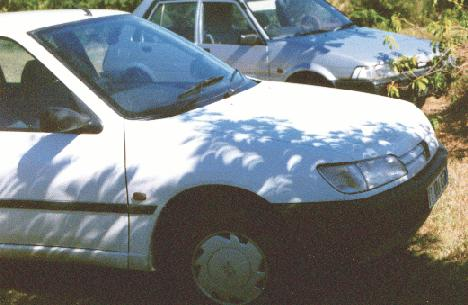Pinhole images on a car during the 1998 eclipse in Guadeloupe.

It's really important that you don't look at the Sun directly, not even while it is being eclipsed, as this can seriously damage your eyes. Even sunglasses aren't good enough protection. But even if you haven't got specially produced eclipse viewing glasses, chances are there is something in your house that will help you view it. A colander and a piece of paper, for example. Standing with your back to the Sun, let the light pass through the colander onto the paper to see lots of images of the eclipsed Sun appear. You could also use a mirror to reflect the light onto a wall, or build your own pinhole camera. See this lovely booklet, produced by the Royal Astronomical Society, to find out more.

Now all we need is a clear sky!

March 17, 2015

Were you stuck in traffic on your way to work or school this morning? Then you might take consolation in the thought of yourself as a tiny experimental subject in the giant petri dish of the UK's motorways, contributing your data points to a greater understanding of traffic flow.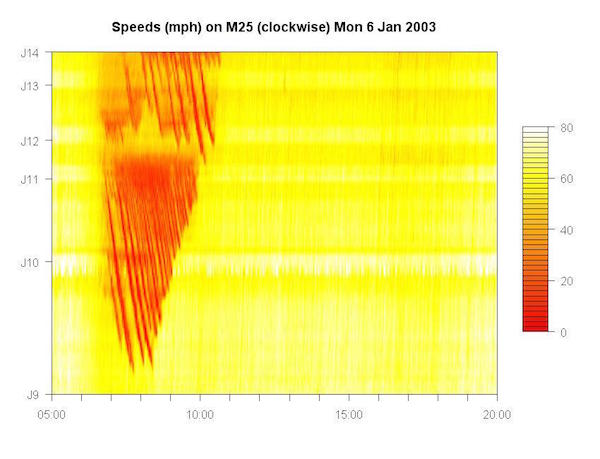Our image of the week shows a heat map of the traffic on a clockwise section of the M25 (junction 9 to junction 14) showing the speed of cars as a colour, from pale yellow for around 80 mph to red for stationary, as they pass along this stretch of road on a typical Monday. The image was created by Richard Gibbons, from the University of Cambridge's Computer Laboratory. It is based on data collected by the Highways Agency from MIDAS (the Motorway Incident Detection and Automatic Signalling) system, archived minute by minute since 1997 at loop detectors every few hundred metres under many UK motorways.

The diagonal red stripes streaking down the image from the left to right between 7am and 11am are driver's commuting pain manifested in data. A driver's path through the data will start at the bottom and move upwards as they passed along the motorway and to the right as time passed. And if they passed junction 9 at, say, 7.30 in the morning, they would have hit red stripe after red stripe, like striking the incoming wave fronts as you try to swim out from a beach.

These stripes are the wave fronts of a stop and go wave, the infuriating pattern of repeated stopping (red) and starting (yellow) that is well known to both motorists and traffic modellers. This is a kind of shock wave that moves back through the traffic, opposite to the direction the cars are travelling. This can be easily seen in the heat map: one starts around junction 12 and moving backwards down the motorway as time goes on.

Click here to see previous images of the week.

March 14, 2015

Today, written as 3/14 the American way, is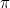day! This special day happens every year, but today is extra special! It’s 3/14/15, giving us the first five digits of that lovely number, rather than just three! But why should we care about all these digits?

If you had a sloppy maths teacher at school you might have grown up with the idea that the numberis equal to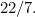Now that is completely wrong. Writing those numbers out in decimal gives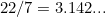while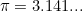. There’s a difference in the third decimal place after the decimal point!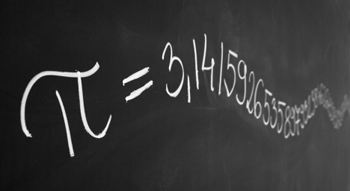How accurately do we need to know the value of π?

Surely this small inaccuracy doesn't matter? Well, as the following extract from a longer article by Chris Budd shows, it really does.

The point is thatis not any number. It lies at the heart of any technology that involves rotation or waves, and that is much of mechanical and electrical engineering. If rotating parts in, say, a typical jet engine are not manufactured to high tolerance, then the parts simply won’t rotate. This typically involves measurements correct to one part in 10,000 and, as these measurements involve, we require a value ofto at least this order of accuracy to prevent errors. In medical imaging using CAT or MRI scanners, the scanning devices move on a ring which has to be manufactured to a tolerance of one part in 1,000,000, requiring an even more precise value of.

However, even this level of accuracy pales into insignificance when we look at modern electrical devices. In high frequency electronics, with frequencies in the order of 1GHz (typical for mobile phones or GPS applications), electrical engineers require a precision in the value used forin the order of one part in 1,000,000,000,000,000.

So, the modern world needsand it needs it accurately!